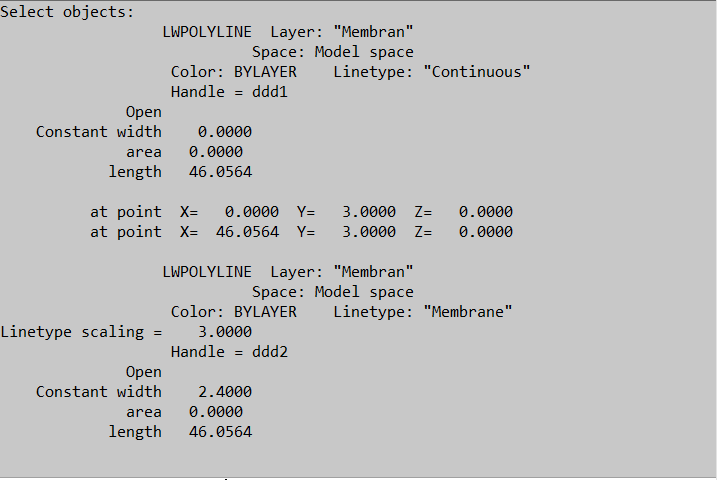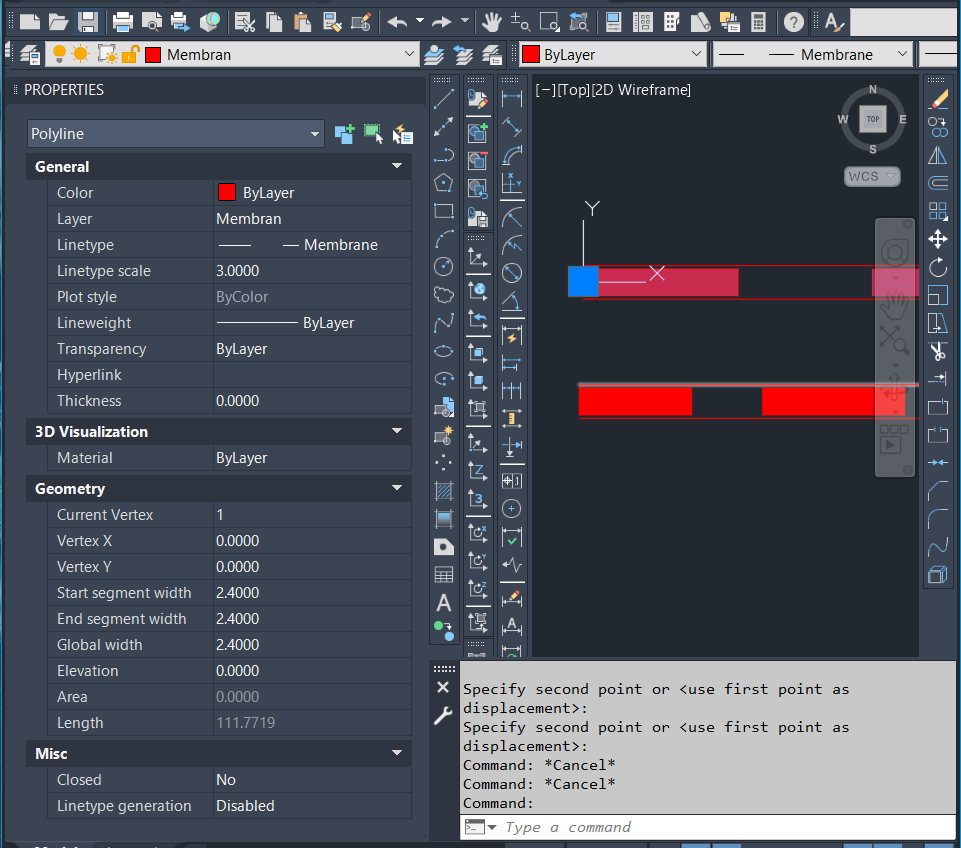# FEATURED POLYLINE

Hello,
I want to draw a polyline with the features I want with the command. My specs are attached. I tried to write an existing lisp, but because I'm very new, I couldn't get the result I wanted.
Could you help.(defun c:pline_to_Membrane()
(command "_.PLINE" (setq sset (ssget "X" (list (cons 0 "PLINE")))))
(setq sset (ssget '(62 "0,255,255")))
(princ))

• Something like

(defun c:plpts ( / pt)
(setvar 'plinewid (getreal "\nEnter width "))
(setq pt (getpoint "\nStarting point of Pline : "))
(command "_pline" pt)
(while (= (getvar "cmdactive") 1 )
(command (getpoint (getvar 'lastpoint)))
)
(command "chprop" "L" "" "LT" "Membrane" "S" 3 "")
)

or
(defun c:plpts24 ( / pt)
(setvar 'plinewid 2.4)
(setq pt (getpoint "\nStarting point of Pline : "))
(command "_pline" pt)
(while (= (getvar "cmdactive") 1 )
(command (getpoint (getvar 'lastpoint)))
)
(command "chprop" "L" "" "LT" "Membrane" "S" 3 "")
)
• Actually, that's exactly what I want, and the underlying lisp offset provides it to draw like this. Where is the area where I can add more features to your writing? I also need to enter the line properties. When I run the command, it should draw exactly as in the image. I know I'm asking a lot, I'm sorry. But believe me, when I combined the two, I couldn't get it to work. I may have made the wrong combination.(defun c:pffs (/ doc ly msp ww-ss c ww-say ww-t ww-data ww-layer ww-of1 ww-of2 c)
(setq doc (vla-get-activedocument (vlax-get-acad-object))
ly (vla-get-layers doc)
msp (vla-get-ModelSpace doc))
(if (setq ww-ss (ssget (list (cons 0 "LWPOLYLINE"))))
(progn
(setq c 0
ww-say (sslength ww-ss))
(if (= ww-mes nil)(setq ww-mes 5))
(setq ww-m (getint (strcat "\nwidth < " (rtos ww-mes 2 2) " >:")))
(if (= ww-m nil)(setq ww-m ww-mes))
(setq ww-mes ww-m)

(defun layac (la n1 n2)
(if (= (tblsearch "layer" la) nil)
(setq lya (vla-add ly (strcat ww-layer "-w"))))
(vla-put-layer (vlax-ename->vla-object n1) la)
(vla-put-layer (vlax-ename->vla-object n2) la))
(while (< c ww-say)
(setq ww-t (ssname ww-ss c)
ww-data (vlax-ename->vla-object ww-t)
ww-layer (vla-get-layer ww-data))
(if (vlax-method-applicable-p ww-data 'offset)
(progn
(vla-offset ww-data (/ ww-mes 2.0))
(setq ww-of1 (entlast))
(vla-offset ww-data (- 0 (/ ww-mes 2.0)))
(setq ww-of2 (entlast))
(layac (strcat ww-layer "-w") ww-of1 ww-of2)
(setq htc (vla-addhatch msp acHatchPatternTypePreDefined "SOLID" :vlax-True))
(command ".-hatch" "s" ww-of1 ww-of2 "" "p" "solid" "Layer" (strcat ww-layer "-w") "")))
(setq c (1+ c)))))(princ))

ALANH said:

Something like

(defun c:plpts ( / pt)
(setvar 'plinewid (getreal "\nEnter width "))
(setq pt (getpoint "\nStarting point of Pline : "))
(command "_pline" pt)
(while (= (getvar "cmdactive") 1 )
(command (getpoint (getvar 'lastpoint)))
)
(command "chprop" "L" "" "LT" "Membrane" "S" 3 "")
)

or
(defun c:plpts24 ( / pt)
(setvar 'plinewid 2.4)
(setq pt (getpoint "\nStarting point of Pline : "))
(command "_pline" pt)
(while (= (getvar "cmdactive") 1 )
(command (getpoint (getvar 'lastpoint)))
)
(command "chprop" "L" "" "LT" "Membrane" "S" 3 "")
)

• Have you considered using the tool palette? There you would be able to set properties without writing any code.

(defun c:pl24 ( / oldlt)
(setq oldlt (getvar 'celtype))
(setvar 'celtype "Membrane")
(setvar 'CELTSCALE 3)
(setvar 'plinewid 2.4)
(setq pt (getpoint "\nStarting point of Pline : "))
(command "_pline" pt)
(while (= (getvar "cmdactive") 1 )
(command (getpoint (getvar 'lastpoint)))
)
(setvar 'celtype oldlt)
(setvar 'CELTSCALE 1)
(setvar 'plinewid 0.0)
(princ)
)